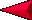Rock Cycle - Minerals (4B) Pre Lab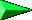OBJECTIVES: Comparing shapes with geometric models. Drawing mineral shapes. VOCABULARY: cubic diamond shaped element mineral pyramid rhombohedron rock tabular MATERIALS: geometric models Students learn to draw geometric shapes.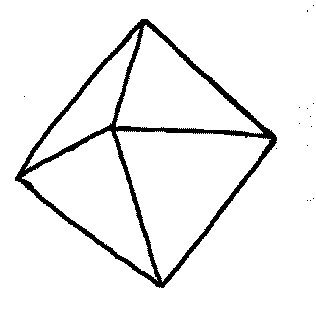A dipyramid
BACKGROUND:

The shape of a mineral crystal reflects the arrangement of atoms which compose it. The nature of this arrangement is affected by the size of the atoms and how they bond together. Mineral breakage is also determined by atomic arrangement; some minerals cleave along weak planes in the atomic structure.

It is important for students to be able to recognize geometric shapes in minerals. Many times geometry and science are taught independently. Therefore, students sometimes do not realize that geometry is a mathematical (or quantitative) way of describing the real world. For example, the shapes of mineral crystals and the mineral cleavage may be described by geometric terms. However, note that real mineral crystals rarely occur as perfect geometric forms.

Shapes help us recognize objects. Mathematicians give names to very specific shapes. This exercise teaches students four common shapes that are commonly seen in minerals.

PROCEDURE:

1. Draw the three geometric shapes on the board. Ask the students to identify them. Illustrate the difference between a square and a cube and between a rectangle and a rhombohedron. Use geometric models if you have them available

2. Have students practice drawing the shapes.

3. Give the students a copy of the quartz crystal model. After they cut it out, make sure the students fold each line before they glue it together. It will make assembly much easier. You may wish to have them color the model before assembling it.

INSTRUCTIONS FOR DRAWING GEOMETRIC SHAPES

CUBIC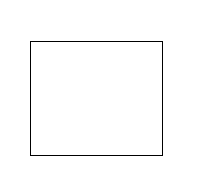1. draw square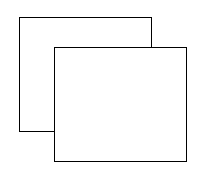2. draw second square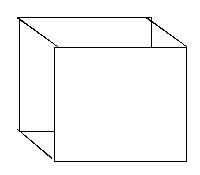3. connect corners

RHOMBOHEDRAL

 1. draw parallelogram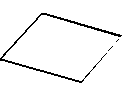2. draw second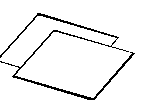3. connect corners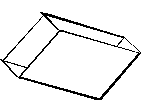TABULAR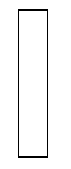1. draw column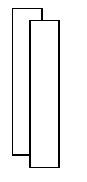2. draw second column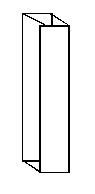3. connect corners[Dictionary]   [Back to Rock Cycle Grid]   [Back to Minerals (4)]